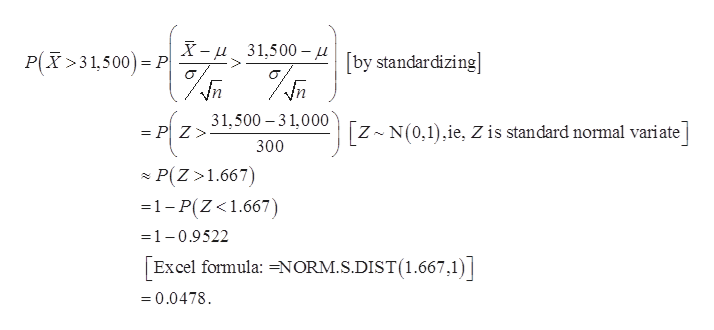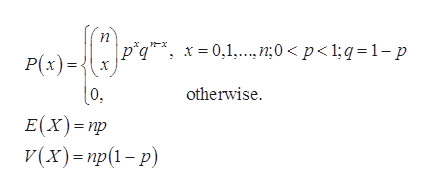How do you know when to use a specific distribution? For example, when would we use the Normal, binomial, grometric, hypergeometric, or negative binomial distribution? Could you give a few examples?

Question

How do you know when to use a specific distribution? For example, when would we use the Normal, binomial, grometric, hypergeometric, or negative binomial distribution? Could you give a few examples?

Step 1

Normal distribution:

Several tests of normality exist, using which you can verify whether a particular data follows the normal distribution.

Usually, before conducting a formal test, we prefer to take the help of graphical methods, to see if the data may be assumed to follow the normal distribution, at least approximately. A few such graphical methods are:

• Histogram of the data , superimposed with a normal probability curve,
• Normal probability plot with confidence interval,
• Normal quantile-quantile (QQ) plot.
• Boxplot, etc.

If the graphical display appears to show at least an approximate normal distribution, then a formal test can be used to verify the normality. A few such tests are as follows:

• Pearson’s Chi-squared test for goodness of fit,
• Shapiro-Wilk test,
• Kolmogorov-Smirnov test, etc.

Assumptions of normality:

The assumptions of normality are as follows:

• The data should be symmetric.
• The data should be mesokurtic.
• The empirical rule must be satisfied.

Empirical rule:

• 68% of values fall within one standard deviation from the mean.
• 95% of values fall within two standard deviations from the mean.
• 99.7% of values fall within three standard deviations from the mean.
Step 2

Example:

Consider the salaries of the elementary school teacher in a particular city with mean value of 31,000 and a standard deviation of 3,000 dollars.

Let X is the salary of a randomly selected elementary school teacher in the United States. It is mentioned that, µ­ = 31,000 and σ = 3,000.

Here, a sample of n = 100 teachers is considered, which is quite a large sample size. So, it may be assumed that the sample mean has an approximate normal distribution with µ­ = 31,000 and σ/√n = 3,000/100 = 300.

The probability that the mean salary of 100 elementary school teachers is greater than \$31,500 can be obtained by standardizing the random variable and using Excel as follows:help_outlineImage TranscriptioncloseX-31,500-[by standardizing P(X>31,500 P PZ31.500 -3 1,000 [z N(0,1),ie, Z is standard normal variate 300 P(Z 1.667) 1-P(Z<1.667) 1-0.9522 Excel formula: =NORM.S.DIST(1.667,1) 0.0478 fullscreen
Step 3

Binomial distribution:

The binomial distribution gives the probability of number of successes out of n trials in a series of Bernoulli trial...help_outlineImage Transcriptionclosepiqx0,1. n;0 < p<1;q 1-p P(x) otherwise E(x) np (х)- пр(1 - р) fullscreen

Want to see the full answer?

See Solution

Want to see this answer and more?

Our solutions are written by experts, many with advanced degrees, and available 24/7

See Solution
Tagged in

Hypothesis Testing